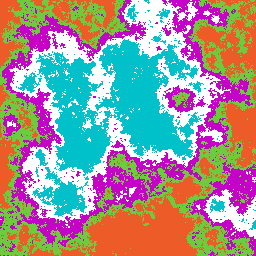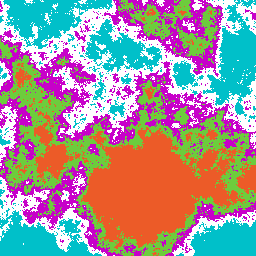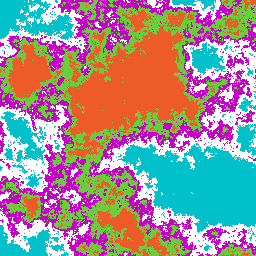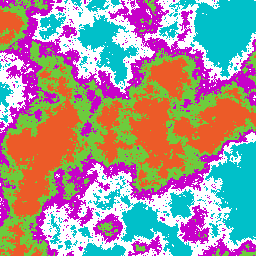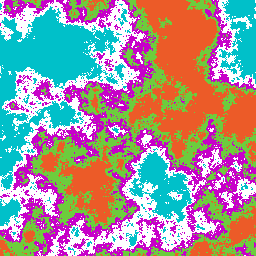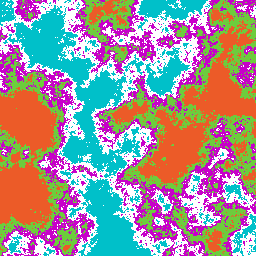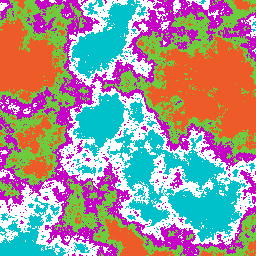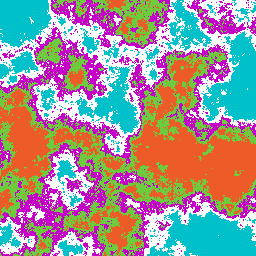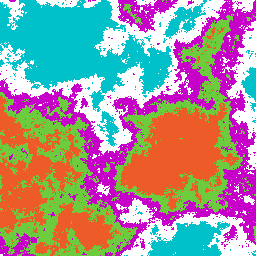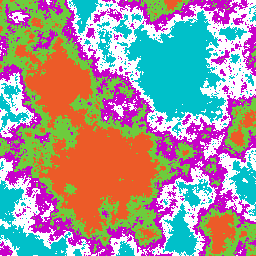### Gaussian and Clipped Gaussian Random Fields

The graphics on this page is based on the PhD thesis of (1) Boris Kozintsev, 1999. Images of isotropic Gaussian random fields (mean 0, variance 1) and their clipped versions can be generated on-line using software created by > (2) Boris Kozintsev, 1999. The on-line implementation requires a correlation structure (4 choices are available; an additional is custom-built), correlation parameters, field size (e.g. 256 by 256), desired output format, and some clipping information.

Clipped Gaussian random fields can be used for modeling discrete-valued random fields with a given correlation structure. If every quantization level is represented by a specific color, then clipping at several levels produces a color map. Note that in "cgi?3" there are 3 colors, in "cgi?9" there are 9 colors, etc.

The following S-Plus image is a 128 by 128 realization of an isotropic Gaussian field with Matern correlation with parameters 5 and 2. The 3D data for similar images for a given isotropic correlation function can be generated on line in the form of triplets (x,y,z) or just the z coordinate, as needed.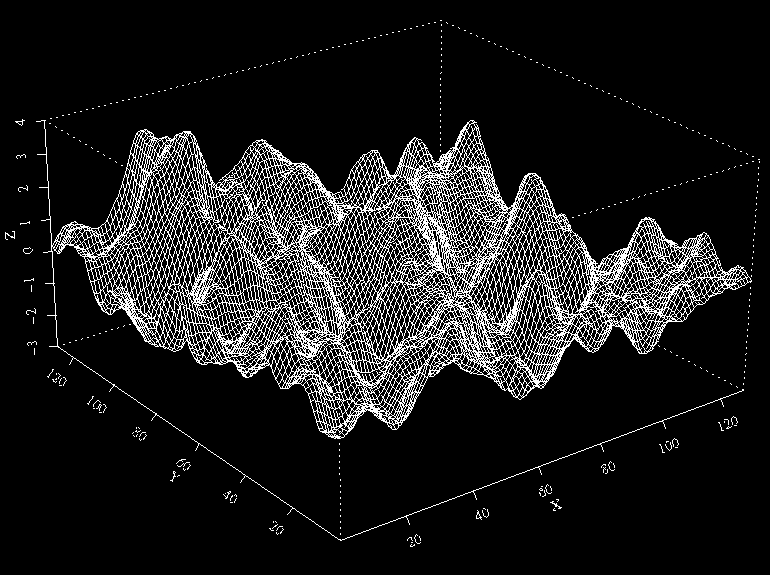A mixure of red and blue adds some color to 128 by 128 Matern(5,5), still using S-Plus,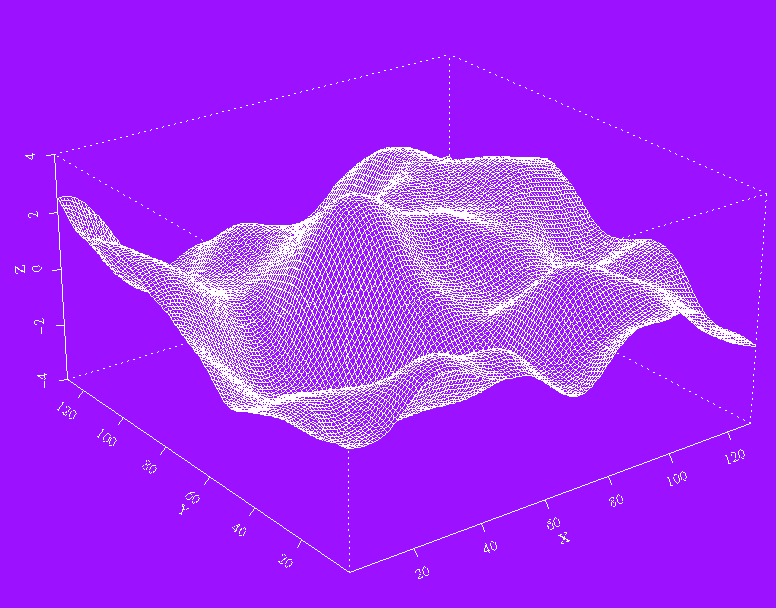Matern(2,5) 128b 128 realization plotted with S-Plus looks like this,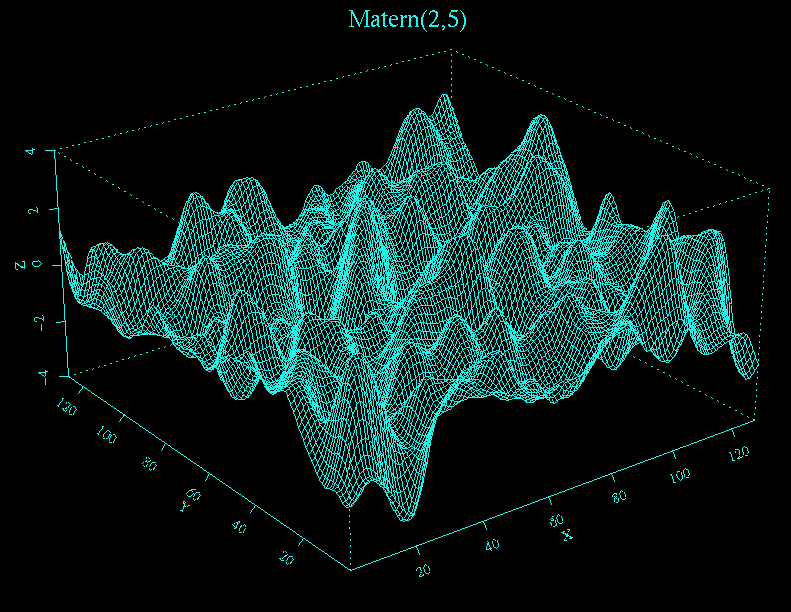A rougher surface is obtained with Matern(2,2) correlation,Switching to Matlab, here is a 128 by 128 realization with Matern(11,2) correlation function plotted with Matlab,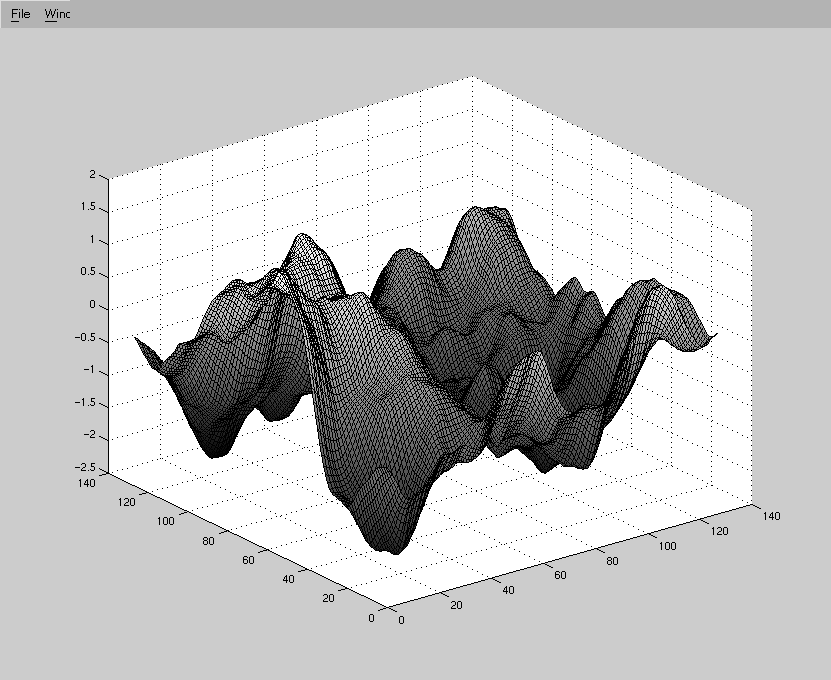The same with random coloring,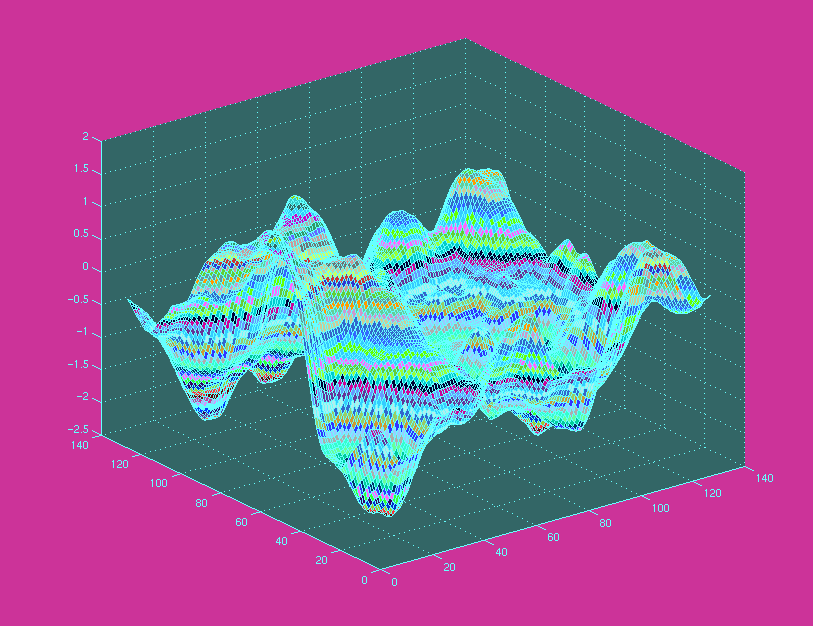Realizations with spherical correlation function tend to be more oscillatory as the following 128 by 128 image with correlation parameter 120.Next is even a rougher 128 by 128 realization from an exponential correlation function with parameters 0.8 and 1.6.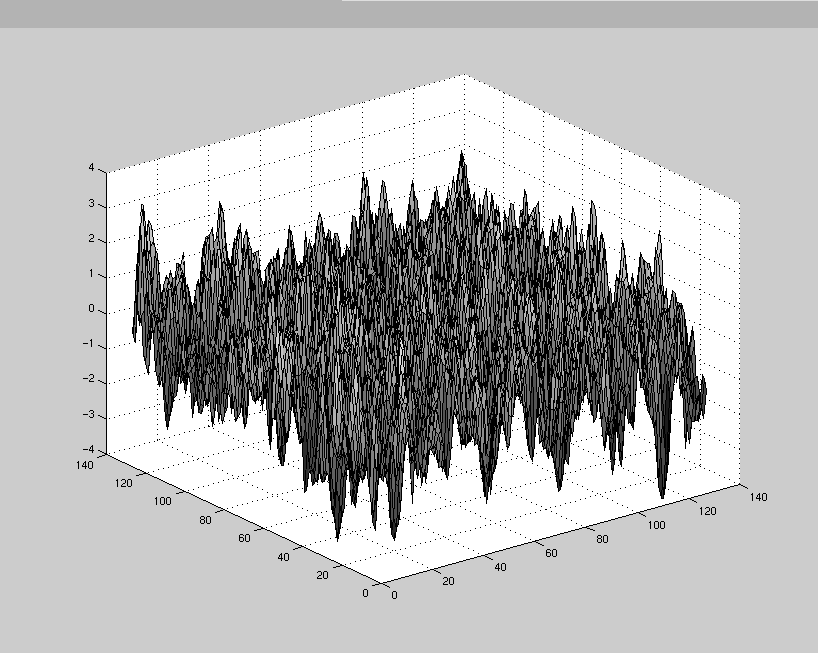A 128 by 128 realization from a rational quadratic correlation function with parameters 4 and 2 looks like this: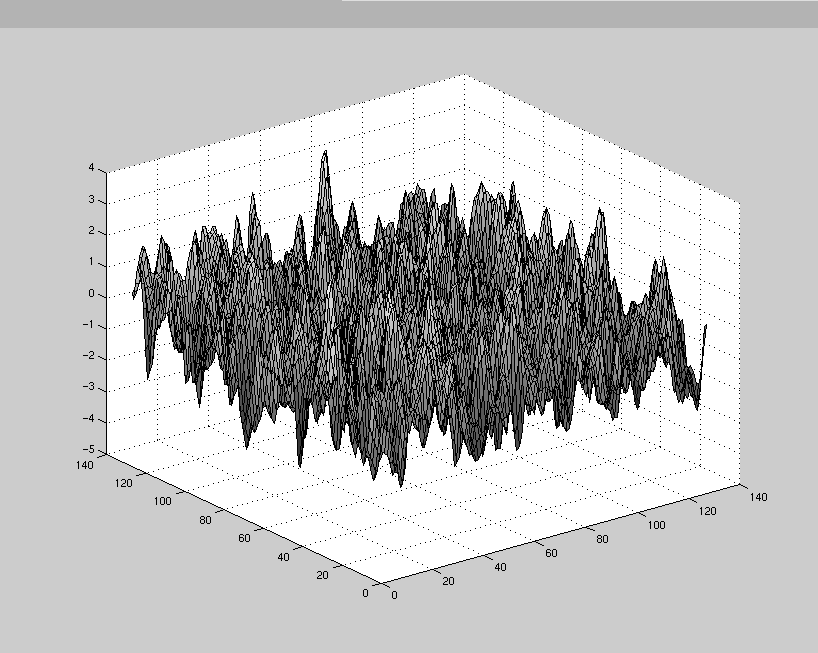Put some color on a 128 by 128 realization from a rational quadratic correlation function with parameters 5 and 2, reverting to S-Plus,Clipping--or quantization--at several levels provides an alternative way to visualize a random field. The following color maps were obtained by clipping at several levels a 256 by 256 Gaussian field with isotropic Matern correlation with parameters 7 and 4, rational quadratic correlation with parameters 10 and 7, and rational quadratic correlation with parameters 2 and 1, respectively.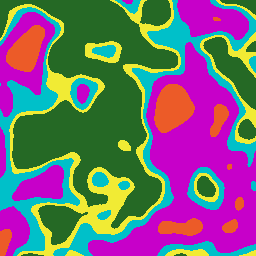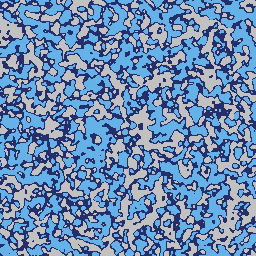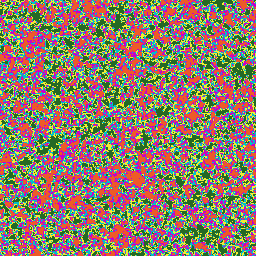Similarly, the next triplet pertain to spherical correlation with parameter 50, exponential correlation with parameters .5 and .9, and Matern correlation with parameters 7 and 2 at 10 levels, respectively.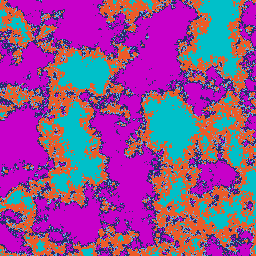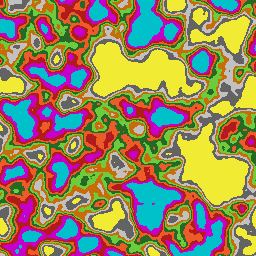The following clipped images were obtained from three realizations of an isotropic Gaussian random field with spherical correlation with parameter 100.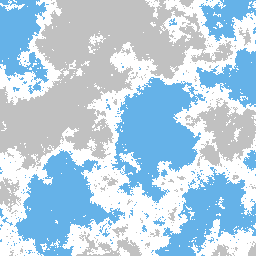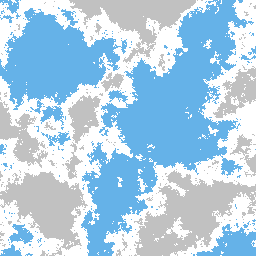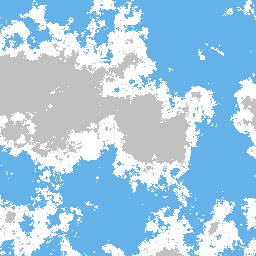The next clipped images were obtained from different realizations of an isotropic Gaussian random field with Matern correlation with parameters 0.9 and 1.9.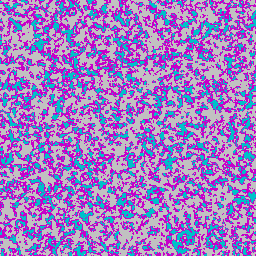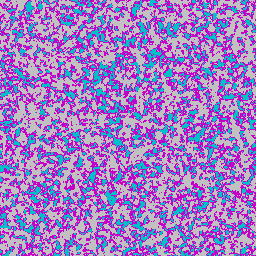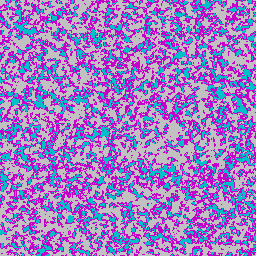Next is a triplet of clipped images corresponding to Matern(5,2), spherical(5), and rational quadratic(5,2).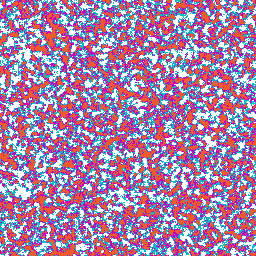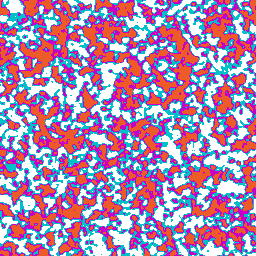Next is a triplet of clipped images--statistically identical--corresponding to spherical(77). 6 colors: light green, orange, magenta, white, cyan, and forest green.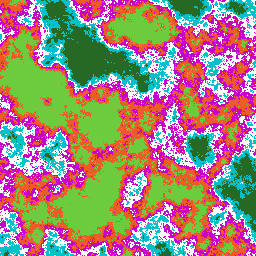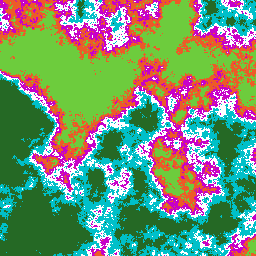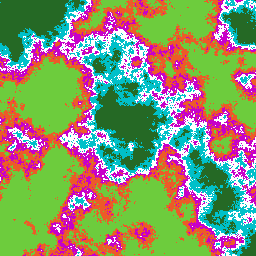Three clipped iamges, 256 by 256, Matern(5,4), 7 colors: orange, magenta, cyan, dark blue, light blue, forest green, yellow.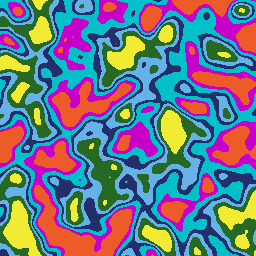Some 256 by 256 binary images with exponential(.8,2) correlation,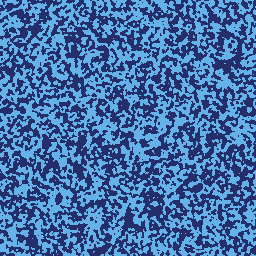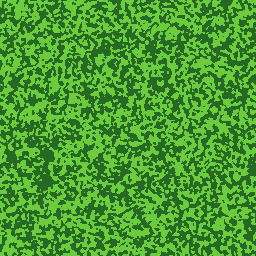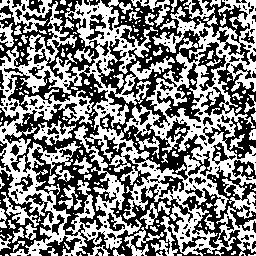Two 300 by 300 images corresponding to spherical(17). 8 colors: dark blue, yellow, brown, magenta, light green, forest green, orange, red, at 12.5% each.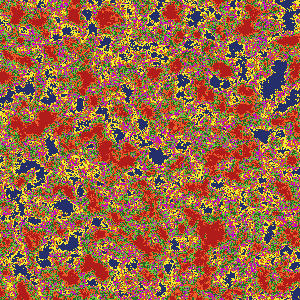Finally, 12 independent images from spherical(120), 5 colors (orange, light green, magenta, white, cyan) at 20% each, each of scaled size 256 by 256.